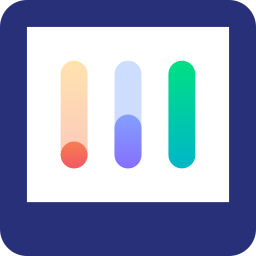## Image Charts

• Category: Open Data

# Image-Charts Public API Documentation

Image-Charts provides a powerful and easy-to-use API for generating charts and graphs on the fly. This API can be integrated with any web application, and it allows developers to easily customize the appearance of charts and graphs according to their needs.

In this blog post, we will explore the various API endpoints provided by Image-Charts and provide examples of how to use them in JavaScript.

## Getting Started

Before we can use the Image-Charts API, we need to create an account and generate an API key. This can be done by visiting the Image-Charts website and following the instructions provided there.

Once we have our API key, we can start generating charts and graphs using the API endpoints.

## API Endpoints

Image-Charts provides a wide range of API endpoints for generating charts and graphs. These endpoints can be used to generate various types of charts, such as bar charts, line graphs, pie charts, and more.

Here are a few examples of API endpoints:

### Basic Bar Chart

To generate a basic bar chart, we can use the following API endpoint:

``````https://image-charts.com/chart?cht=bvg&chs=400x300&chd=t:10,20,30&chxt=x,y&chxl=0:|Jan|Feb|Mar&chxr=1,0,30
``````

This will generate a bar chart with three data points: 10, 20, and 30. The chart will have a width of 400 pixels and a height of 300 pixels. The x-axis will display the months Jan, Feb, and Mar, and the y-axis will range from 0 to 30.

Here's how we can use this endpoint in JavaScript:

``````<img src="https://image-charts.com/chart?cht=bvg&chs=400x300&chd=t:10,20,30&chxt=x,y&chxl=0:|Jan|Feb|Mar&chxr=1,0,30" alt="Basic Bar Chart">
``````

### Line Graph

To generate a line graph, we can use the following API endpoint:

``````https://image-charts.com/chart?cht=lc&chs=400x300&chd=t:10,20,30|20,30,40&chxt=x,y&chxl=0:|Jan|Feb|Mar&chxr=1,0,40
``````

This will generate a line graph with two data sets: [10, 20, 30] and [20, 30, 40]. The chart will have a width of 400 pixels and a height of 300 pixels. The x-axis will display the months Jan, Feb, and Mar, and the y-axis will range from 0 to 40.

Here's how we can use this endpoint in JavaScript:

``````<img src="https://image-charts.com/chart?cht=lc&chs=400x300&chd=t:10,20,30|20,30,40&chxt=x,y&chxl=0:|Jan|Feb|Mar&chxr=1,0,40" alt="Line Graph">
``````

### Pie Chart

To generate a pie chart, we can use the following API endpoint:

``````https://image-charts.com/chart?cht=p&chs=400x300&chd=t:30,20,50&chl=Red|Green|Blue&chco=ff0000,00ff00,0000ff
``````

This will generate a pie chart with three data points: 30, 20, and 50. The chart will have a width of 400 pixels and a height of 300 pixels. The labels for the wedges will be "Red", "Green", and "Blue", and the colors for the wedges will be red, green, and blue, respectively.

Here's how we can use this endpoint in JavaScript:

``````<img src="https://image-charts.com/chart?cht=p&chs=400x300&chd=t:30,20,50&chl=Red|Green|Blue&chco=ff0000,00ff00,0000ff" alt="Pie Chart">
``````

## Conclusion

The Image-Charts API provides an easy way to generate charts and graphs on the fly. By using the API endpoints provided by Image-Charts and customizing them according to our needs, we can easily create charts and graphs for our web applications.

In this blog post, we explored a few examples of API endpoints and how to use them in JavaScript. With these examples as a starting point, we can begin to create more complex and customized charts and graphs using the Image-Charts API.

Visit to Image Charts website